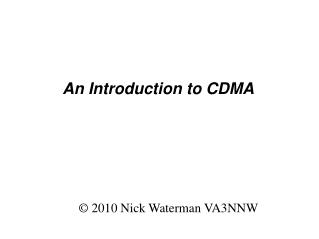DownloadDownload PresentationAn Introduction to CDMA

An Introduction to CDMA

Download PresentationAn Introduction to CDMA

- - - - - - - - - - - - - - - - - - - - - - - - - - - E N D - - - - - - - - - - - - - - - - - - - - - - - - - - -
Presentation Transcript

1. © 2010 Nick Waterman VA3NNW An Introduction to CDMA

2. An Introduction to CDMA • Follows on quite nicely from Mike Jarabek's “Modulation schemes and some light coding theory” talk in November • CDMA is a coding scheme used in many 3G cellular networks • There will be SOME math

3. An Introduction to CDMA • Follows on quite nicely from Mike Jarabek's “Modulation schemes and some light coding theory” talk in November • CDMA is a coding scheme used in many 3G cellular networks • There will be SOME maths

4. An Introduction to CDMA • Follows on quite nicely from Mike Jarabek's “Modulation schemes and some light coding theory” talk in November • CDMA is a coding scheme used in many 3G cellular networks • There will be SOME maths, but quite easy (x and +)

5. CDMA? What does it stand for? • Code • Division • Multiple • Access

6. Multiple Access?

7. FDMA • Frequency • Division • Multiple • Access

8. FDMA

9. FDMA (1G Cellular Networks)

10. TDMA • Time • Division • Multiple • Access

11. TDMA

12. F/TDMA • Frequency and Time • Division • Multiple • Access

13. (2G Cellular Networks) F/TDMA

14. Ham Bands F/TDMA

15. ? CDMA • CODE • Division • Multiple • Access

16. CDMA

17. CDMA Uses the whole band, for the whole time (Scares the ҨӁɜʨѪ out of the CW narrow-bandwidth fans)

18. CDMA Everyone transmits on top of everyone else. How do we show this?

19. CDMA Some people show it like this... … but I prefer

20. CDMA (3G Cellular Networks)

21. … but you're probably wondering...

22. Hang on, Everyone transmits on top of each other? Across the whole band? At the same time?

23. YES. With different codes, and if you know the right code, you can receive just the person transmitting with that code.

24. Err, OK... So how do we make it? First, a bit of a reminder: AM and SSB

25. Frequency Spectrum

26. Voice

27. Voice, Carrier → … and mix ...

28. AM … or SSB ...

29. SSB

30. Let's do the same for CDMA:

31. Data Signal … and a very wideband spreading code ...

32. Data Signal + Code → … and mix ...

33. CDMA … to make really wideband CDMA spread spectrum signal … but we can also turn the power down ...

34. CDMA … right down ...

35. CDMA … below the noise floor! … and still be heard! Trust me. We'll prove it later.

36. … and the hardware? What does that look like? Let's again compare with SSB:

37. SSB Transceiver Block Diagram

38. CDMA Transceiver Block Diagram Except this is usually mostly digital, so...

39. CDMA Transceiver Block Diagram

40. Can we walk through an example?

41. WARNING! Here comes the maths! … but it's quite simple multiplication … though it does include negative numbers. Don't worry, my 8-year old daughter can do it :-)

42. Multiplication 1 x 1 = 1 1 x -1 = -1 -1 x 1 = -1 -1 x -1 = 1

43. CDMA example Low-Bandwidth Signal: High-Bandwidth Spreading Code: ...repeated...

44. CDMA example Low-Bandwidth Signal: High-Bandwidth Spreading Code: Mix is a simple multiply … and transmit.

45. CDMA example To Decode / Receive, take the signal:

46. CDMA example To Decode / Receive, take the signal: Multiply by the same Spreading Code: … to get ... … which you should recognise as...

47. CDMA example To Decode / Receive, take the signal: Multiply by the same Spreading Code: … to get ...

48. (Discuss noise) To Decode / Receive, take the signal: Multiply by the same Spreading Code: … to get ...

49. What if we use the wrong code? Take the same signal: Multiply by the wrong Spreading Code:

50. What if we use the wrong code? Take the same signal: Multiply by the wrong Spreading Code: … for example, let's just shift the same code left a bit: